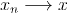Analysis WebNotes
arrow_back arrow_forward

# Class Contents

## Sequences

In this chapter we shall begin to study convergence. The main type of convergence we shall consider is convergence of sequences of real numbers.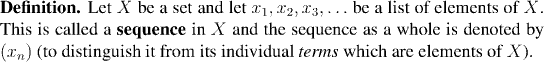Sequences can be defined in many ways: the two most common ways are sequences defined by formulas and sequences defined recursively. Nevertheless, when we discuss sequences there is no particular reason to think of them arising in any one way. Fundamentally, a sequence is just a function from the natural numbers into the set X (usually the real numbers, in this chapter).

Example:

The sequence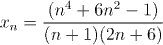is an example of a sequence defined by a formula.

Example:

The sequence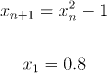is an example of a sequence defined by recursion (alternatively, we say it is defined inductively).

## Distance Between Real Numbers

If the idea of convergence is that the terms of a sequence are getting closer and closer to some fixed number, then this has to do with distance, and we need to be clear about how to measure distance between real numbers.

Factoid:

If x and y are real numbers then the distance between them is |x-y|.

## Convergence of Sequences

Loosely speaking: A sequence converges to the limit a of, as we pass along the sequence, the terms get closer and closer to a. This is the idea one wants to keep firmly in mind as we look at the next couple of examples, and, especially, when we come to the formal definition of convergence.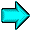Click here to run an animated demonstration of convergence

Example:

Consider the sequence:

```   x_1=1
x_2=1.4
x_3=1.41
x_4=1.414                                             (note)
x_5=1.4142
x_6=1.41421
```
where, in general, x_n is root 2 expressed to the first n significant places. Clearly x_n is getting closer and closer to root 2, without ever reaching it.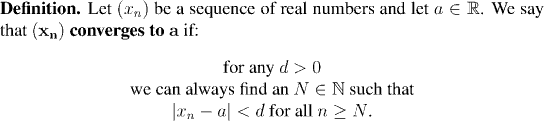Example:

Let x_n=1/n. Then (x_n) converges to zero.

Example:

Let x_n=1/2^n (note). Then (x_n) converges to zero.

In this last example, the thing we really used was that 1/2^n < 1/n. We can make this the basis of a useful lemma: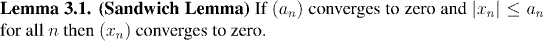In fact, knowing what sequences converge to zero is very useful, since sequences which converge to zero can be made the basis of our entire understanding of limits. The following lemma makes this explicit: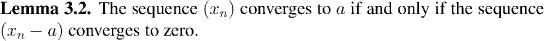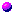Notation:

Since sequences which converge to zero are so useful, we have a special name for the set of all sequences which converge to zero; we call it c_0. So, when a sequence converges to zero, we say that it belongs to c_0.

Example:

Let x_n=(n+2)/(n+1). Then (x_n) converges to 1.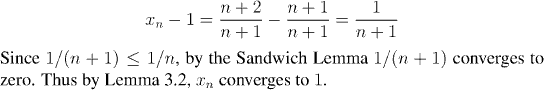Note:

This last example draws attention to the fact that even quite simple limits call for the use of lemmas and theorems to evaluate. It would be possible to work out the proof of the last limit from first principles (and probably instructional---look for examples like this in coming homework and Lab) but when you want to know the answer in practice, you look for results which help you simplify the sequence. We shall pick up this theme in a little while.

If (x_n) converges to (ie gets closer and closer to) a number a then it doesn't seem reasonable that it should converge to some other number at the same time. And, indeed, a convergent sequence can only converge to one number, but there is nothing in the definition of convergence which explicitly says that the number a is the only number which relates to (x_n) in that particular way. We need to prove this: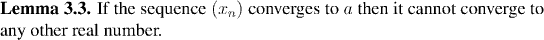Notation:

Since a convergent sequence can only converge to one possible number, we call this number the limit of the sequence, and write: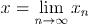or